Theory and Modern Applications

# On ${L}_{p}\left(k\right)$-equivalence of impulsive differential equations and its applications to partial impulsive differential equations

## Abstract

By means of the Schauder-Tychonoff principle, ${L}_{p}\left(k\right)$-equivalence are established between linear and nonlinear perturbed impulsive differential equations with an unbounded linear part in an arbitrary Banach space. The feasibility of our theoretical results is illustrated by an example involving partial impulsive differential equations of the parabolic type.

MSC:34A37, 35Q72, 47H10.

## 1 Introduction

Theory of impulsive differential equations has been emerging as an important area of investigation since these equations provide natural frameworks for describing many real life phenomena appearing in physics, chemical technology, population dynamics, and economics; see the remarkable monographs . There have been significant contributions regarding the investigations of qualitative properties of solutions of such equations in the last three decades . However, dealing with nonlinear impulsive differential equations have faced, as usual, several drawbacks that caused slackening progress of this theory.

One of the most important ways to investigate the asymptotic structure of two equations is to build asymptotic equivalence between their solutions. Establishing such equivalence enables researchers to comment on the asymptotic behavior of solutions of certain nonlinear equations by studying another linear equation whose solutions bear the same character. In spite of the fact that this way is of great significance in the theory of analysis, it has been less considered in the literature.

In this paper, we introduce the definition of ${L}_{p}\left(k\right)$-equivalence between linear and nonlinear perturbed impulsive differential equations with an unbounded linear part in an arbitrary Banach space. By means of the Schauder-Tychonoff principle, sufficient conditions are established to guarantee the existence of such equivalence. To expose the feasibility of our theoretical results, an example involving partial impulsive differential equations of the parabolic type is provided. Similar problems but with different approaches regarding ${L}_{p}\left(k\right)$-equivalence for impulsive differential equations were first reported in the papers .

## 2 Statement of the problem

Let $\mathbb{X}$ be a Banach space with the norm $\parallel \cdot \parallel$ and the identical operator I. By $D\left(T\right)\subset \mathbb{X}$, we will denote the domain of the operator $T:D\left(T\right)\to \mathbb{X}$.

Consider the following two impulsive differential equations:

$\left\{\begin{array}{c}\frac{d{u}_{1}}{dt}=A\left(t\right){u}_{1},\phantom{\rule{1em}{0ex}}t\ne {t}_{n},\hfill \\ {u}_{1}\left({t}_{n}^{+}\right)={Q}_{n}\left({u}_{1}\left({t}_{n}\right)\right),\phantom{\rule{1em}{0ex}}n=1,2,\dots ,\hfill \end{array}$
(1)

and

$\left\{\begin{array}{c}\frac{d{u}_{2}}{dt}=A\left(t\right){u}_{2}+f\left(t,{u}_{2}\right),\phantom{\rule{1em}{0ex}}t\ne {t}_{n},\hfill \\ {u}_{2}\left({t}_{n}^{+}\right)={Q}_{n}\left({u}_{2}\left({t}_{n}\right)\right)+{h}_{n}\left({u}_{2}\left({t}_{n}\right)\right),\phantom{\rule{1em}{0ex}}n=1,2,\dots ,\hfill \end{array}$
(2)

where $A\left(t\right):D\left(A\left(t\right)\right)\to \mathbb{X}$ (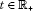) and ${Q}_{n}:D\left({Q}_{n}\right)\to D\left(A\left({t}_{n}\right)\right)$ are linear and possibly unbounded operators. The functions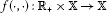and ${h}_{n}:\mathbb{X}\to \mathbb{X}$ are continuous where ${\mathbb{R}}_{+}=\left[0,\mathrm{\infty }\right)$. The sets $D\left(A\left(t\right)\right)$ and $D\left({Q}_{n}\right)$ are dense in $\mathbb{X}$. The points of jumps ${t}_{n}$ satisfy the conditions $0={t}_{0}<{t}_{1}<{t}_{2}<\cdots <{t}_{n}<\cdots$ with ${lim}_{n\to \mathrm{\infty }}{t}_{n}=\mathrm{\infty }$. We set ${Q}_{0}=I$ and ${h}_{0}\left(u\right)=0$ ($u\in \mathbb{X}$).

Furthermore, we assume that all functions under consideration are left continuous and that there exists the Cauchy operator $U\left(t,s\right)$ ($0\le s\le t$) of the linear equation

$\frac{d{u}_{1}}{dt}=A\left(t\right){u}_{1}.$
(3)

Sufficient conditions for the existence of $U\left(t,s\right)$ can be found in [18, 19]. One can easily check that

$V\left(t,s\right)=U\left(t,s\right){Q}_{n}U\left({t}_{n},{t}_{n-1}\right){Q}_{n-1}\cdots {Q}_{k}U\left({t}_{k},s\right)\phantom{\rule{1em}{0ex}}\left(0\le s\le {t}_{k}<{t}_{n}
(4)

is the Cauchy operator of the linear impulsive differential equation (1). We observe that the operator $V\left(t,s\right)$ is bounded if one of the following conditions hold:

(B1) ${Q}_{n}U\left({t}_{n},{t}_{n-1}\right)$ are bounded operators, $n=1,2,\dots$ .

(B2) $U\left({t}_{n+1},{t}_{n}\right){Q}_{n}$ are bounded operators, $n=1,2,\dots$ .

Lemma 1 Let one of the conditions (B 1) or (B 2) hold. Then the solution $u\left(t\right)$ of the equation

$u\left(t\right)=V\left(t,0\right)u\left(0\right)+{\int }_{0}^{t}V\left(t,s\right)f\left(s,u\left(s\right)\right)\phantom{\rule{0.2em}{0ex}}ds+\sum _{0<{t}_{n}
(5)

satisfies the impulsive differential equation (2).

The proof of the above statement is straightforward and can be achieved by direct substitution.

Let the following condition be fulfilled:

(H) There exists continuous function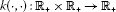such that

$\parallel V\left(t,s\right)\xi \parallel \le k\left(t,s\right)\parallel \xi \parallel ,$

where $0\le s and $\xi \in D\left(A\left(s\right)\right)$.

We introduce the following spaces: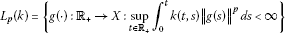and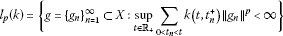with the norms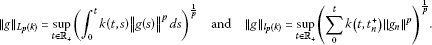The following conditions are needed in the sequel:

(H1) There exists constant ${M}_{1}>0$ such that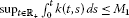.

(H2) There exists constant ${M}_{2}>0$ such that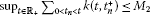.

Definition 1 Equation (2) is called ${L}_{p}\left(k\right)$-equivalent to Eq. (1) in the nonempty, closed, and convex subset B of $\mathbb{X}$ if there exists a convex and closed subset D of $\mathbb{X}$ such that for any solution ${u}_{1}\left(t\right)$ of (1) lying in the set B there exists a solution ${u}_{2}\left(t\right)$ of (2) lying in the set $B\cup D$, and satisfying the relation ${u}_{2}\left(t\right)-{u}_{1}\left(t\right)\in {L}_{p}\left(k\right)$.

If Eq. (2) is ${L}_{p}\left(k\right)$-equivalent to Eq. (1) in the set B, and vice versa, we say that Eqs. (1) and (2) are ${L}_{p}\left(k\right)$-equivalent in the set B.

By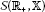, we denote the linear set of all functions which are continuous for $t\ne {t}_{n}$ ($n=1,2,\dots$), having left and right limits at points ${t}_{n}$ and are left continuous. The setis a locally convex space with respect to the metric

$\rho \left(u,v\right)=\underset{0

The convergence with respect to this metric coincides with the uniform convergence on each bounded interval. For this space, an analogue of Arzella-Ascoli’s theorem is valid.

Lemma 2 ()

The set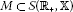is relatively compact if the intersections $M\left(t\right)=\left\{m\left(t\right):m\in M\right\}$ are relatively compact forand M is equicontinuous on each interval $\left({t}_{n},{t}_{n+1}\right]$, $n=0,1,2,\dots$.

The proof of the above theorems is completed by applying the theorem of Arzella-Ascoli on each interval $\left({t}_{n},{t}_{n+1}\right]$, $n=0,1,2,\dots$ and constitutes a diagonal line sequence.

Let C be a nonempty subset of X. Set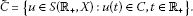We now have the following lemma.

Lemma 3 ()

Let C be a nonempty, convex, and closed subset of $\mathbb{X}$. Suppose an operator T transforms $\stackrel{˜}{C}$ into itself and is continuous and compact. Then T has a fixed point in $\stackrel{˜}{C}$.

The proof of Lemma 3 follows by utilizing the fixed-point principle of Schauder-Tychonoff.

The following lemmas are borrowed from .

Lemma 4 ()

Let the following conditions be fulfilled:

1. 1.

Condition (B 1) (or (B 2)) holds.

2. 2.

Conditions (H), (H 1), and (H 2) hold.

Then, for any function $F\in {L}_{p}\left(k\right)$ and for any sequence $H={\left\{{H}_{n}\right\}}_{n=1}^{\mathrm{\infty }}\in {l}_{p}\left(k\right)$, the linear nonhomogeneous impulsive differential equation

$\left\{\begin{array}{c}\frac{du}{dt}=A\left(t\right)u+F\left(t\right),\phantom{\rule{1em}{0ex}}t\ne {t}_{n},\hfill \\ u\left({t}_{n}^{+}\right)={Q}_{n}\left(u\left({t}_{n}\right)\right)+{H}_{n}\left(u\left({t}_{n}\right)\right),\phantom{\rule{1em}{0ex}}n=1,2,\dots ,\hfill \end{array}$
(6)

has a bounded solution $u\left(t\right)$ for which

$u\left(t\right)=V\left(t,0\right)u\left(0\right)+{\int }_{0}^{t}V\left(t,s\right)F\left(s\right)\phantom{\rule{0.2em}{0ex}}ds+\sum _{0<{t}_{n}
(7)

and

$\parallel u\left(t\right)\parallel \le \parallel V\left(t,0\right)u\left(0\right)\parallel +{M}_{1}^{\frac{1}{q}}{\parallel F\parallel }_{{L}_{p}\left(k\right)}+{M}_{2}^{\frac{1}{q}}{\parallel H\parallel }_{{l}_{p}\left(k\right)}.$
(8)

Lemma 5 ()

Let the following conditions be fulfilled:

1. 1.

Condition (B 1) (or (B 2)) holds.

2. 2.

Conditions (H) and (H 1) hold.

Then the operator ${G}_{1}$ defined by the formula

${G}_{1}F\left(t\right)={\int }_{0}^{t}V\left(t,s\right)F\left(s\right)\phantom{\rule{0.2em}{0ex}}ds$
(9)

maps ${L}_{p}\left(k\right)$ into ${L}_{p}\left(k\right)$ and the following estimate is valid:

${\parallel {G}_{1}F\parallel }_{{L}_{p}\left(k\right)}\le {M}_{1}{\parallel F\parallel }_{{L}_{p}\left(k\right)},$
(10)

where $\frac{1}{p}+\frac{1}{q}=1$.

Lemma 6 ()

Let the following conditions be fulfilled:

1. 1.

Condition (B 1) (or (B 2)) holds.

2. 2.

Conditions (H) and (H 2) hold.

Then the operator ${G}_{2}$, defined by the formula

${G}_{2}H\left(t\right)=\sum _{0<{t}_{n}
(11)

maps ${l}_{p}\left(k\right)$ into ${L}_{p}\left(k\right)$ and the following estimate is valid:

${\parallel {G}_{2}H\parallel }_{{L}_{p}\left(k\right)}\le {M}_{1}^{\frac{1}{p}}{M}_{2}^{\frac{1}{q}}{\parallel H\parallel }_{{l}_{p}\left(k\right)},$
(12)

where $\frac{1}{p}+\frac{1}{q}=1$.

## 3 The main results

Set

$u\left(t\right)={u}_{2}\left(t\right)-{u}_{1}\left(t\right),$

where ${u}_{1}\left(t\right)$ is the solution of Eq. (1) and ${u}_{2}\left(t\right)$ is the solution of Eq. (2).

Then ${u}_{1}\left(t\right)=V\left(t,0\right){u}_{1}\left(0\right)$ and ${u}_{2}\left(t\right)$ is solution of the linear nonhomogeneous impulsive differential equation (6) for $F\left(t\right)=f\left(t,{u}_{2}\left(t\right)\right)$ and ${H}_{n}={h}_{n}\left({u}_{2}\left({t}_{n}\right)\right)$. Consequently,

$u\left(t\right)={G}_{1}f\left(t,{u}_{1}\left(t\right)+u\left(t\right)\right)+{G}_{2}{h}_{n}\left({u}_{1}\left({t}_{n}\right)+u\left({t}_{n}\right)\right).$

We define the operator

$T\left({u}_{1},u\right)\left(t\right)={G}_{1}f\left(t,{u}_{1}\left(t\right)+u\left(t\right)\right)+{G}_{2}{h}_{n}\left({u}_{1}\left({t}_{n}\right)+u\left({t}_{n}\right)\right).$
(13)

Theorem 1 Let the following conditions be fulfilled:

1. 1.

Condition (B 1) (or (B 2)) holds.

2. 2.

Conditions (H), (H 1), and (H 2) hold.

3. 3.

There exists a nonempty, convex, and closed subset D of X such that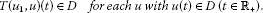4. 4.

For any fixed ${u}_{1}\in \stackrel{˜}{B}$, the following inclusions hold:

4.1 ${\int }_{0}^{t}V\left(t,s\right)f\left(s,{u}_{1}\left(s\right)+u\left(s\right)\right)\phantom{\rule{0.2em}{0ex}}ds\in {K}^{{u}_{1}}\left(t\right)$ ($u\in \stackrel{˜}{D}$),

4.2 ${\sum }_{0<{t}_{n} ($u\in \stackrel{˜}{D}$), where ${K}^{{u}_{1}}\left(t\right)$ is for any fixedand ${K}_{n}^{{u}_{1}}$ is for any fixed $n=1,2,\dots$ a compact subset of X.

1. 5.

${sup}_{w\in \stackrel{˜}{B}\cup \stackrel{˜}{D}}\parallel f\left(t,w\right)\parallel \le F\left(t\right)$, for, where the function $F\left(t\right)$ is continuous and $F\in {L}_{p}\left(k\right)$.

2. 6.

${sup}_{w\in \stackrel{˜}{B}\cup \stackrel{˜}{D}}{h}_{n}\left(w\right)\le {H}_{n}$, for $n=1,2,\dots$, where the sequence $H={\left\{{H}_{n}\right\}}_{n=1}^{\mathrm{\infty }}\in {l}_{p}\left(k\right)$.

Then Eq. (2) is ${L}_{p}\left(k\right)$-equivalent to Eq. (1) in the set B and the following estimate is valid:

${\parallel {u}_{2}-{u}_{1}\parallel }_{{L}_{p}\left(k\right)}\le {M}_{1}{\parallel F\parallel }_{{L}_{p}\left(k\right)}+{M}_{1}^{\frac{1}{p}}{M}_{2}^{\frac{1}{q}}{\parallel H\parallel }_{{l}_{p}\left(k\right)}.$

Proof We will prove that for each solution ${u}_{1}\left(t\right)$ of Eq. (1) lying in the set B the operator $T\left({u}_{1},u\right)$ has a fixed point $u\left(t\right)$ such that ${u}_{1}+u\in \stackrel{˜}{B}\cup \stackrel{˜}{D}$ and lies in ${L}_{p}\left(k\right)$.

In view of condition 3 of Theorem 1, it follows that the operator $T\left({u}_{1},u\right)$ defined by (13) maps the set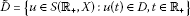into itself for ${u}_{1}\in \stackrel{˜}{B}$.

Let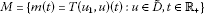. We will show the equicontinuity of the functions in the set M. Let ${t}^{\mathrm{\prime }}>{t}^{\mathrm{\prime }\mathrm{\prime }}$ and ${t}^{\mathrm{\prime }},{t}^{\mathrm{\prime }\mathrm{\prime }}\in \left({t}_{n},{t}_{n+1}\right]$. It can be verified that

$\begin{array}{rcl}\parallel m\left({t}^{\mathrm{\prime }}\right)-m\left({t}^{\mathrm{\prime }\mathrm{\prime }}\right)\parallel & \le & \underset{w\in \stackrel{˜}{B}\cup \stackrel{˜}{D}}{sup}{\int }_{0}^{{t}^{\mathrm{\prime }\mathrm{\prime }}}\parallel V\left({t}^{\mathrm{\prime }},s\right)-V\left({t}^{\mathrm{\prime }\mathrm{\prime }},s\right)\parallel \parallel f\left(s,w\right)\parallel \phantom{\rule{0.2em}{0ex}}ds\\ +\underset{w\in \stackrel{˜}{B}\cup \stackrel{˜}{D}}{sup}{\int }_{{t}^{\mathrm{\prime }\mathrm{\prime }}}^{{t}^{\mathrm{\prime }}}\parallel V\left({t}^{\mathrm{\prime }},s\right)\parallel \parallel f\left(s,w\right)\parallel \phantom{\rule{0.2em}{0ex}}ds\\ +\underset{w\in \stackrel{˜}{B}\cup \stackrel{˜}{D}}{sup}\sum _{0<{t}_{n}<{t}^{\mathrm{\prime }\mathrm{\prime }}}\parallel V\left({t}^{\mathrm{\prime }},{t}_{n}^{+}\right)-V\left({t}^{\mathrm{\prime }\mathrm{\prime }},{t}_{n}^{+}\right)\parallel \parallel {h}_{n}\left(w\right)\parallel .\end{array}$

The continuity of the function $V\left(t,s\right)$ on $\left({t}_{n},{t}_{n+1}\right]$, condition (H) and condition 5 of Theorem 1 imply the equicontinuity of the set M.

From condition 4 of Theorem 1 and (13), we deduce the compactness of the intersections $M\left(t\right)=\left\{m\left(t\right):m\in M\right\}$ for. Consequently, from Lemma 2, it follows that the set M is compact.

We will show that the operator $T\left({u}_{1},u\right)$ is continuous in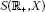. Let the sequence ${\left\{{\stackrel{˜}{u}}_{k}\right\}}_{k=1}^{\mathrm{\infty }}\subset \stackrel{˜}{D}$ be convergent to the function $\stackrel{˜}{u}\in D$ in the metric of the space.

Then the sequence

${\left\{{z}_{k}\left(t\right)\right\}}_{k=1}^{\mathrm{\infty }}={\left\{f\left(t,{u}_{1}\left(t\right)+{\stackrel{˜}{u}}_{k}\left(t\right)\right)\right\}}_{k=1}^{\mathrm{\infty }}$

tends to $z\left(t\right)=f\left(t,{u}_{1}\left(t\right)+\stackrel{˜}{u}\left(t\right)\right)$ for anyand the sequence

${\left\{{v}_{k}\left(n\right)\right\}}_{k=1}^{\mathrm{\infty }}={\left\{{h}_{n}\left({u}_{1}\left({t}_{n}\right)+{\stackrel{˜}{u}}_{k}\left({t}_{n}\right)\right)\right\}}_{k=1}^{\mathrm{\infty }}$

tends to ${\left\{v\left(n\right)\right\}}_{n=1}^{\mathrm{\infty }}={\left\{{h}_{n}\left({u}_{1}\left({t}_{n}\right)+\stackrel{˜}{u}\left({t}_{n}\right)\right)\right\}}_{n=1}^{\mathrm{\infty }}$ coordinate-wisely ($n=1,2,\dots$).

From conditions 5 and 6 of Theorem 1, it follows that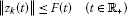and

$\parallel {v}_{k}\left(n\right)\parallel \le {H}_{n}\phantom{\rule{1em}{0ex}}\left(n=1,2,\dots \right).$

In virtue of the theorem of Lebesgue, we take the limit inside the integral and obtain that the sequence of functions ${G}_{1}{z}_{k}\left(t\right)$ tends forto the function ${G}_{1}z\left(t\right)$. On the other hand, by the analogue of the theorem of Lebesgue for the series, we obtain that the sequence of functions ${G}_{2}\left({v}_{k}\left(n\right)\right)\left(t\right)$ tends to the function ${G}_{2}\left(v\left(n\right)\right)\left(t\right)$. Since the functions

${G}_{1}{z}_{k}\left(t\right)+{G}_{2}\left({v}_{k}\left(n\right)\right)\left(t\right)$

lie in a compact set, they also tend to the metric of the space.

In view of Lemma 3, it follows that for any ${u}_{1}\in \stackrel{˜}{B}$ the operator $T\left({u}_{1},u\right)$ has a fixed point $u\in \stackrel{˜}{D}$, that is, $T\left({u}_{1},u\right)=u$.

From conditions 5 and 6 of Theorem 1, Lemma 5, and Lemma 6, it follows that this fixed point $u\in {L}_{p}\left(k\right)$ and the following estimate is valid:

${\parallel u\parallel }_{{L}_{p}\left(k\right)}\le {M}_{1}{\parallel F\parallel }_{{L}_{p}\left(k\right)}+{M}_{1}^{\frac{1}{p}}{M}_{2}^{\frac{1}{q}}{\parallel H\parallel }_{{l}_{p}\left(k\right)},$

where $\frac{1}{p}+\frac{1}{q}=1$. □

Remark 1 The case when the operator $A\left(t\right)$ () is linear bounded and the sets B and D are balls is considered in .

We shall illustrate the effectiveness of Theorem 1 by constructing an example involving the partial impulsive differential equations. For more details on the general theory of partial impulsive differential equations, we suggest that the reader consults [20, 21].

## 4 An example

In this section, we consider linear and nonlinear perturbed partial impulsive differential equations. We transform these equations to ordinary impulsive differential equations with an unbounded linear operator and show that they satisfy the conditions of Theorem 1. More information regarding the theory of ordinary differential equations with an unbounded linear operator can be reached at .

Let Ω be a bounded domain with smooth boundary Ω in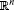, $Q=\left(0,\mathrm{\infty }\right)×\mathrm{\Omega }$, and $\mathrm{\Gamma }=\left(0,\mathrm{\infty }\right)×\partial \mathrm{\Omega }$.

We denote

${P}_{n}=\left\{\left({t}_{n},x\right):x\in \mathrm{\Omega }\right\},\phantom{\rule{2em}{0ex}}P=\bigcup _{n=1}^{\mathrm{\infty }}{P}_{n}$

and

${\mathrm{\Lambda }}_{n}=\left\{\left({t}_{n},x\right):x\in \partial \mathrm{\Omega }\right\},\phantom{\rule{2em}{0ex}}\mathrm{\Lambda }=\bigcup _{n=1}^{\mathrm{\infty }}{\mathrm{\Lambda }}_{n}.$

Consider the following linear impulsive parabolic initial value problem:

$\left\{\begin{array}{c}\frac{\partial {u}_{1}}{\partial t}=\stackrel{˜}{A}\left(t,x,D\right){u}_{1},\phantom{\rule{1em}{0ex}}\left(t,x\right)\in Q\setminus P,\hfill \\ {D}^{\alpha }{u}_{1}\left(t,x\right)=0,\phantom{\rule{1em}{0ex}}|\alpha |
(14)

and the nonlinear perturbed impulsive parabolic initial value problem

$\left\{\begin{array}{c}\frac{\partial {u}_{2}}{\partial t}=\stackrel{˜}{A}\left(t,x,D\right){u}_{2}+\stackrel{˜}{f}\left(t,x,{u}_{2}\right),\phantom{\rule{1em}{0ex}}\left(t,x\right)\in Q\setminus P,\hfill \\ {D}^{\alpha }{u}_{2}\left(t,x\right)=0,\phantom{\rule{1em}{0ex}}|\alpha |
(15)

where $\stackrel{˜}{A}\left(t,x,D\right)={\sum }_{|\alpha |\le 2m}{a}_{\alpha }\left(t,x\right){D}^{\alpha }$, ${\stackrel{˜}{Q}}_{n}:D\left({\stackrel{˜}{Q}}_{n}\right)\to D\left(\stackrel{˜}{A}\left({t}_{n},x,D\right)\right)$ ($n=1,2,\dots$) are linear operators and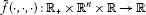and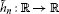are continuous functions.

Let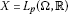($1\le p<\mathrm{\infty }$), where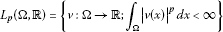with the norm ${|v|}_{p}={\left({\int }_{\mathrm{\Omega }}{|v\left(x\right)|}^{p}\phantom{\rule{0.2em}{0ex}}dx\right)}^{\frac{1}{p}}$.

Along with the family $\stackrel{˜}{A}\left(t,x,D\right)$,of strongly elliptic operators, we associate a family of linear operators $A\left(t\right)$,acting in X by

This is can be achieved as follows: $D=D\left(A\left(t\right)\right)={W}^{2m,p}\left(\mathrm{\Omega }\right)\cap {W}_{0}^{m,p}\left(\mathrm{\Omega }\right)$,.

Let ${v}_{i}\in X$ ($i=1,2$). We set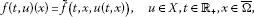and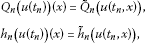where ${Q}_{n}:D\left({Q}_{n}\right)\to D$, $D\left({Q}_{n}\right)\subset X$ lie dense in X and are linear operators,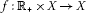and ${h}_{n}:X\to X$ are continuous functions.

We claim that Eqs. (1) and (2) are ${L}_{p}\left(k\right)$-equivalent. Let $U\left(t,s\right)$ be the Cauchy operator of the linear equation (3).

Sufficient conditions for the validity of the estimate

can be found in .

Let ${t}_{n}=n$ ($n=1,2,\dots$), $\stackrel{˜}{f}\left(t,x,{u}_{2}\right)={e}^{-\gamma t}sin{u}_{2}\left(t,x\right)$, ${\stackrel{˜}{h}}_{n}\left({u}_{2}\left({t}_{n},x\right)\right)={e}^{-\alpha n}\frac{1}{1+{u}_{2}^{2}\left({t}_{n},x\right)}$ and ${\stackrel{˜}{Q}}_{n}\xi =\frac{{e}^{-{k}_{1}}}{C}{q}_{n}\left(n\right)\xi$ (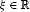), where the positive constants α, γ, and ${k}_{1}$ satisfy $k+{k}_{1}-\alpha p>0$, $k+{k}_{1}-\gamma p>0$ and ${k}_{1}>{2}^{\frac{1}{p}}C-k$. The functions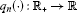($n=1,2,\dots$) are defined such that $|{q}_{n}\left(t\right)|\le 1$ for each.

Then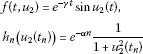and

${Q}_{n}\eta =\frac{{e}^{-{k}_{1}}}{C}{q}_{n}\left(n\right)\eta \phantom{\rule{1em}{0ex}}\left(\eta \in X\right).$

We shall find the function $k\left(t,s\right)$ ($0\le s\le t$) of condition (H). Let $0 and $\xi \in D$. Then the following estimate is valid

$\begin{array}{rcl}{|V\left(t,s\right)\xi |}_{p}& =& {|U\left(t,{t}_{n}\right){Q}_{n}\cdots {Q}_{m}U\left({t}_{m},s\right)\xi |}_{p}\\ \le & C{e}^{-k\left(t-n\right)}\frac{{e}^{-{k}_{1}}}{c}{q}_{n}\left(n\right)\cdots \frac{{e}^{-{k}_{1}}}{c}{q}_{m}\left(m\right)C{e}^{-k\left(m-s\right)}{|\xi |}_{p}\\ \le & C{e}^{-k\left(t-s\right)}{e}^{-{k}_{1}\left(n-m+1\right)}{|\xi |}_{p}\\ \le & C{e}^{-\left(k+{k}_{1}\right)\left(t-s\right)}{|\xi |}_{p}.\end{array}$

Set

$k\left(t,s\right)=C{e}^{-\left(k+{k}_{1}\right)\left(t-s\right)}\phantom{\rule{1em}{0ex}}\left(0\le s\le t\right).$

For the constant ${M}_{1}$ of condition (H1), we obtain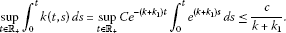Hence,

${M}_{1}=\frac{c}{k+{k}_{1}}.$

For the constant ${M}_{2}$ of condition (H2), we obtain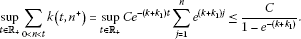Hence,

${M}_{2}=\frac{C}{1-{e}^{-\left(k+{k}_{1}\right)}}.$

Let $r>0$ and

$\rho >\frac{{2}^{\frac{1}{p}}C\left(k+{k}_{1}\right)}{k+{k}_{1}-{2}^{\frac{1}{p}}C}\left(\frac{r}{k+{k}_{1}}+\frac{{\mu }^{\frac{1}{p}}\left(\mathrm{\Omega }\right)}{1-{e}^{\alpha p-k-{k}_{1}}}\right).$
(16)

We shall find the function ${F}_{r+\rho }\left(t\right)$ and the sequence ${H}_{r+\rho }={\left\{{H}_{n,r+\rho }\right\}}_{n=1}^{\mathrm{\infty }}$ so that conditions 5 and 6 of Theorem 1 are satisfied. We observe that

$\underset{{|w|}_{p}\le r+\rho }{sup}{|f\left(t,w\right)|}_{p}=\underset{{|w|}_{p}\le r+\rho }{sup}{e}^{-\gamma t}{|sinw|}_{p}\le {e}^{-\gamma t}\left(r+\rho \right)$

and

$\underset{{|w|}_{p}\le r+\rho }{sup}{|{h}_{n}\left(w\right)|}_{p}=\underset{{|w|}_{p}\le r+\rho }{sup}{e}^{-\alpha n}{|\frac{1}{1+{w}^{2}}|}_{p}\le {e}^{-\alpha n}{\mu }^{\frac{1}{p}}\left(\mathrm{\Omega }\right).$

Hence, ${F}_{r+\rho }\left(t\right)={e}^{-\gamma t}\left(r+\rho \right)$ and ${H}_{n,r+\rho }={e}^{-\alpha n}{\mu }^{\frac{1}{p}}\left(\mathrm{\Omega }\right)$.

It remains to show that ${F}_{r+\rho }\in {L}_{p}\left(k\right)$ and ${H}_{r+\rho }\in {l}_{p}\left(k\right)$. Indeed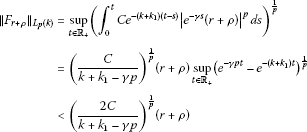and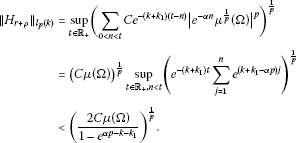Set ${B}_{\sigma }=\left\{u\in X:{|u|}_{p}\le \sigma \right\}$. We shall show that for any ${u}_{1}\left(t\right)\in {B}_{r}$ (), the operator $T\left({u}_{1},u\right)$ defined by (13) maps the set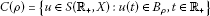into itself.

From (8), (13), and (16), we obtain

$\parallel T\left(u,{u}_{1}\right)\left(t\right)\parallel \le {M}_{1}^{\frac{1}{q}}{\parallel {F}_{r+\rho }\parallel }_{{L}_{p}\left(k\right)}+{M}_{2}^{\frac{1}{q}}{\parallel {H}_{r+\rho }\parallel }_{{l}_{p}\left(k\right)}<\rho$

for each. Hence, the operator $T\left({u}_{1},u\right)$ maps the set $C\left(\rho \right)$ into itself.

By means of a compactness criterion in , we shall prove condition 4.1. We observe that the set

$M\left(t\right)=\left\{m\left(t\right)={\int }_{0}^{t}V\left(t,s\right)f\left(s,{u}_{1}\left(s\right)+u\left(s\right)\right)\phantom{\rule{0.2em}{0ex}}ds:{|u|}_{p}\le \rho ,t\in {\mathbb{R}}^{+}\right\}$

is compact subset of X for any fixed. Indeed

$\begin{array}{rcl}|m\left(t\right)\left(x\right)|& \le & {\int }_{0}^{t}C{e}^{-\left(k+{k}_{1}\right)\left(t-s\right)}{e}^{-\gamma s}|sin\left({u}_{1}\left(s\right)\left(x\right)+u\left(s\right)\left(x\right)\right)|\phantom{\rule{0.2em}{0ex}}ds\\ \le & C{e}^{-\left(k+{k}_{1}\right)t}{\int }_{0}^{t}{e}^{\left(k+{k}_{1}-\gamma \right)s}\phantom{\rule{0.2em}{0ex}}ds\le \frac{2C}{k+{k}_{1}-\gamma }\end{array}$
(17)

and

${|m\left(t\right)\left(x\right)|}_{p}={\left({\int }_{\mathrm{\Omega }}{|m\left(t\right)\left(x\right)|}^{p}\phantom{\rule{0.2em}{0ex}}dx\right)}^{\frac{1}{p}}\le \frac{2C}{k+{k}_{1}-\gamma }{\mu }^{\frac{1}{p}}\left(\mathrm{\Omega }\right)$
(18)

and hence ${|m\left(t\right)\left(x\right)|}_{p}\le N$, where N is a positive constant.

We shall show that

${|m\left(t\right)\left(x+h\right)-m\left(t\right)\left(x\right)|}_{p}\to 0\phantom{\rule{1em}{0ex}}\left(h\to 0\right).$

This follows from the relations below

$\begin{array}{rcl}{|m\left(t\right)\left(x+h\right)-m\left(t\right)\left(x\right)|}_{p}& \le & C{e}^{-\left(k+{k}_{1}\right)t}{\int }_{0}^{t}{e}^{\left(k+{k}_{1}-\gamma \right)s}|sin\left({u}_{1}\left(s\right)\left(x+h\right)+u\left(s\right)\left(x+h\right)\right)\\ -sin\left({u}_{1}\left(s\right)\left(x\right)+u\left(s\right)\left(x\right)\right){|}_{p}\phantom{\rule{0.2em}{0ex}}ds\\ \le & C{e}^{-\left(k+{k}_{1}\right)t}{\int }_{0}^{t}{e}^{\left(k+{k}_{1}-\gamma \right)s}{|{u}_{1}\left(s\right)\left(x+h\right)-{u}_{1}\left(s\right)\left(x\right)|}_{p}\phantom{\rule{0.2em}{0ex}}ds\\ +C{e}^{-\left(k+{k}_{1}\right)t}{\int }_{0}^{t}{e}^{\left(k+{k}_{1}-\gamma \right)s}{|u\left(s\right)\left(x+h\right)-u\left(s\right)\left(x\right)|}_{p}\phantom{\rule{0.2em}{0ex}}ds.\end{array}$

In a similar way, one can show the validity of condition 4.2. The conditions of Theorem 1 are fulfilled, and hence Eqs. (1) and (2) are in ${L}_{p}\left(k\right)$-equivalent. Hence, every solution ${u}_{1}\left(t,x\right)$ of (14) induces a solution ${u}_{2}\left(t,x\right)$ of (15) such that the function $\alpha \left(t\right)=|{u}_{1}\left(t,x\right)-{u}_{2}\left(t,x\right)|$ lies in ${L}_{p}\left(k\right)$ for any $x\in \mathrm{\Omega }$.

## References

1. Lakshmikantham V, Bainov DD, Simeonov PS: Theory of Impulsive Differential Equations. World Scientific, Singapore; 1989.

2. Bainov DD, Covachev V: Impulsive Differential Equations with a Small Parameter. World Scientific, Singapore; 1994.

3. Samoilenko AM, Perestyuk NA: Differential Equations with Impulse Effect. World Scientific, Singapore; 1995.

4. Ahmad S, Stamov G: On the almost periodic processes in impulsive competitive systems with delay and impulsive perturbations. Nonlinear Anal., Real World Appl. 2009, 10(5):2857–2863. 10.1016/j.nonrwa.2008.09.003

5. Ahmad S, Stamov GT: Almost periodic solutions of n -dimensional impulsive competitive systems. Nonlinear Anal., Real World Appl. 2009, 10(3):1846–1853. 10.1016/j.nonrwa.2008.02.020

6. Akhmet MU, Alzabut JO, Zafer A: Perron’s theorem for linear impulsive differential equations with distributed delay. J. Comput. Appl. Math. 2006, 193(1):204–218. 10.1016/j.cam.2005.06.004

7. Allegretto W, Papini D, Forti M: Common asymptotic behavior of solutions and almost periodicity for discontinuous delayed and impulsive networks. IEEE Trans. Neural Netw. 2010, 21(7):1110–1125.

8. Alzabut JO, Nieto JJ, Stamov GT: Existence and exponential stability of positive almost periodic solutions for a model of hematopoiesis. Bound. Value Probl. 2009., 2009: Article ID 127510

9. Bin L, Xinzhi L, Xiaoxin L: Robust stability of uncertain dynamical systems. J. Math. Anal. Appl. 2004, 290(2):519–533. 10.1016/j.jmaa.2003.10.035

10. Alwan MS, Liu X, Xie W: Existence, continuation, and uniqueness problems of stochastic impulsive systems with time delay. J. Franklin Inst. 2010, 347(7):1317–1333. 10.1016/j.jfranklin.2010.06.005

11. Zou L, Xiong Z, Shu Z: The dynamics of an eco-epidemic model with distributed time delay and impulsive control strategy. J. Franklin Inst. 2011, 348(9):2332–2349. 10.1016/j.jfranklin.2011.06.023

12. Li X, Rakkiyappan R, Balasubramaniam P: Existence and global stability analysis of equilibrium of fuzzy cellular neural networks with time delay in the leakage term under impulsive perturbations. J. Franklin Inst. 2011, 348(2):135–155. 10.1016/j.jfranklin.2010.10.009

13. Bainov DD, Kostadinov S, Zabreiko P:${L}_{p}$-equivalence of impulsive equations. Int. J. Theor. Phys. 1988, 27(11):1411–1424. 10.1007/BF00671319

14. Georgieva A:Properties of ${L}_{p}\left(k\right)$-solutions of linear nonhomogeneous impulsive differential equations with unbounded linear operator. Anniversary International Conference REMIA 2010, 129–135.

15. Georgieva A, Kostadinov S:${L}_{p}$-equivalence between a linear and a nonlinear perturbed impulse differential equations with bounded linear part. J. Tech. Univ. Plovdiv, Fundam. Sci. Appl., Ser. A, Pure Appl. Math. 2007, 12: 33–42.

16. Georgieva A, Kostadinov S:${L}_{p}$-equivalence between two nonlinear impulse differential equations with unbounded linear parts and its application for partial impulse differential equations. Rostock. Math. Kolloqu. 2009, 64: 55–66.

17. Georgieva A, Kostadinov S:Sufficient conditions for the ${L}_{p}$-equivalence between two nonlinear impulse differential equations. Turk. J. Math. 2008, 32: 451–466.

18. Krasnoselski, M, Zabreiko, P, Pustilnic, E, Sobolevski, P: Integrable Operators in Spaces of Integrable Functions. Moscow (1966) (in Russian)

19. Pazy A: Semigroups of Linear Operators. Springer, Berlin; 1983.

20. Erbe L, Freedman H, Liu X, Wu J: Comparison principle for impulsive parabolic equations with applications to models of single species growth. J. Aust. Math. Soc. Ser. B 1991, 32: 382–400. 10.1017/S033427000000850X

21. Xiao J, Nieto JJ: Variational approach to some damped Dirichlet nonlinear impulsive differential equations. J. Franklin Inst. 2011, 348(2):369–377. 10.1016/j.jfranklin.2010.12.003

22. Maurin K: Metody Przestrzeni Hilberta. Panstwowe Wydawnictwo Naukove, Warsaw; 1959. in Russian

## Acknowledgements

The authors would like to express their sincere thanks to the editor, Professor Dr. Sandra Pinelas, for handling our paper during the reviewing process and to the referees for suggesting some corrections that helped make the contents of the paper more accurate.

## Author information

Authors

### Corresponding author

Correspondence to JO Alzabut.

### Competing interests

The authors declare that they have no competing interests.

### Authors’ contributions

The authors have achieved equal contributions to each part of this paper. All authors read and approved the final version of the manuscript.

## Rights and permissions

Reprints and Permissions

Georgieva, A., Kostadinov, S., Stamov, G. et al. On ${L}_{p}\left(k\right)$-equivalence of impulsive differential equations and its applications to partial impulsive differential equations. Adv Differ Equ 2012, 144 (2012). https://doi.org/10.1186/1687-1847-2012-144

• ${L}_{p}\left(k\right)$-equivalence